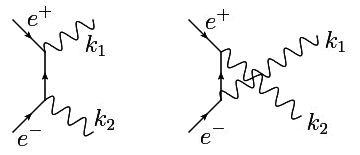# Electron-positron pair annihilation

Consider the following u-channel diagram (on the right).Let the polarization vectors of $k_1$ and $k_2$ be $(\epsilon^{\mu}_{1})^{*}$ and $(\epsilon^{\mu}_{2})^{*}$ respectively.

Do you label the incoming momentum of the positron as $p^{\mu}$ or $p^{\nu}$? In other words, do you associate the incoming positron with the photon $k_1$ or photon $k_2$?

I'm not exactly sure what you're asking. We don't really associate the incoming positron/electron with one of the photons.

If it's just a question of labelling, you'd simply label your incoming $e^+$ and $e^-$ with momenta $p_1$ and $p_2$ respectively and then use momentum conservation at each vertex while following the arrows to label the momentum of the internal line. I've taken the diagram you're interested in and done just that. (note the convention is to draw the arrow of an incoming antiparticle as going out of its vertex, labelling it as $-p_2$). (I've implicitly taken both photon arrows to be pointing outward).If you're question concerns actually computing the factor corresponding to this Feynman diagram, this is given by the Feynman rules. The result is obtained following the fermion (straight line), beginning from the end segment which is pointing out of a vertex (in this case $e^+$), at each point writing down from left to right the appropriate factor for each element (vertex, line) in the diagram, until we reach the other end segment (in this case $e^-$):

$$e^2 \bar{v}_{s_2} \gamma_{\mu} \epsilon^{\mu}_2 \Delta_F(p_1-k_1) \gamma_{\nu}\epsilon^{\nu}_1 u_{s_1}$$

Where $v_{s_2}=v_{s_2}(p_2)$ and $u_{s_1}=u_{s_1}(p_1)$ are Dirac spinors with spins $s_1$ and $s_2$ respectively corresponding to $e⁻$ and $e⁺$, $\epsilon^{\mu}_2 = \epsilon^{\mu}_2(k_2)$ and $\epsilon^{\mu}_1 = \epsilon^{\mu}_1(k_1)$ are the polarization vectors for the photons and $\Delta_F(p_1-k_1)$ is the Feynman fermion propagator associated to the internal fermion line.

So as you can see the polarization vectors are contracted with the gamma matrices $\gamma_{\mu}$ which are independent of momenta.

If I have missed the point of your question or you are not familiar with some elements of my answer please let me know and I will try to resolve your doubts. If you wish I can attach the full list of Feynman rules for QED.